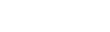# In this article, we will understand the Hull-White model and also do Simulations with QuantLib Python.Mansoor Ahmed

3 months ago | 2 min read
Follow### Introduction

The Hull-White model is financial modeling in Python. It is an ideal of future interest rates in financial mathematics. It is right to the class of no-arbitrage models. Those are capable of appropriate to the latest term structure of interest rates in its best generic development.

The Hull-White model is comparatively direct to translate the mathematical description of the progress of future interest rates onto a tree or frame. Therefore, the interest rate derivatives for example Bermudan swaptions may be valued in the model.

The first Hull-White model was labeled by John C. Hull and Alan White in 1990. That is quite widespread in the market nowadays.

In this article, we will understand the Hull-White model and also do Simulations with QuantLib Python.

### Description

We can define the Hull-White Short Rate Model as:

There is a degree of uncertainty among practitioners about exactly that parameters in the model are time-dependent. Similarly, what name to spread over to the model in each case? The most usually known naming convention is the following:

•  has t (time) dependence that is the Hull-White model.
•  And  are both time-dependent — the long Vasicek model.

We use QuantLib to display how to simulate the Hull-White model and examine some of the properties. We import the libraries and set things up as described below:

``import QuantLib as qlimport matplotlib.pyplot as pltimport numpy as np% matplotlib inline``
• We use the constant for this instance is all well-defined as described below.
• Variables sigma and are the constants that define the Hull-White model.
• We discretize the time span of length thirty years into 360 intervals.
• This is defined by the timestep variable in the simulation.
• We would use a constant forward rate term structure as an input for ease.
• It is the right way to swap with another term structure here.
``sigma = 0.1a = 0.1timestep = 360length = 30 # in yearsforward_rate = 0.05day_count = ql.Thirty360()todays_date = ql.Date(15, 1, 2015)``
``ql.Settings.instance().evaluationDate = todays_datespot_curve = ql.FlatForward(todays_date, ql.QuoteHandle(ql.SimpleQuote(forward_rate)), day_count)spot_curve_handle = ql.YieldTermStructureHandle(spot_curve)``
``hw_process = ql.HullWhiteProcess(spot_curve_handle, a, sigma)rng = ql.GaussianRandomSequenceGenerator(ql.UniformRandomSequenceGenerator(timestep, ql.UniformRandomGenerator()))seq = ql.GaussianPathGenerator(hw_process, length, timestep, rng, False)``
• The Hull-White process is built bypassing the term structure, a and sigma.
• One has to make available a random sequence generator along with other simulation inputs for example timestep and `length to create the path generator.
• A function to make paths may be written as demonstrated below:
``def generate_paths(num_paths, timestep):    arr = np.zeros((num_paths, timestep+1))    for i in range(num_paths):        sample_path = seq.next()        path = sample_path.value()        time = [path.time(j) for j in range(len(path))]        value = [path[j] for j in range(len(path))]        arr[i, :] = np.array(value)    return np.array(time), arr``
• The simulation of the short rates appearance is as follows:
``num_paths = 10time, paths = generate_paths(num_paths, timestep)for i in range(num_paths):    plt.plot(time, paths[i, :], lw=0.8, alpha=0.6)plt.title("Hull-White Short Rate Simulation")plt.show()``

### Monte-Carlo simulation

• On the other hand, valuing vanilla instruments for example caps and swaptions is valuable mainly for calibration.
• The actual use of the model is to value rather more exotic derivatives for example Bermudan swaptions on a lattice.
• Also, other derivatives in a multi-currency context for example Quanto Constant Maturity Swaps.
• These are explained for instance in Brigo and Mercurio (2001).
• The well-organized and precise Monte-Carlo simulation of the Hull-White model with time-dependent parameters may be easily performed.

For more details visit:https://www.technologiesinindustry4.com/2022/01/the-hull-white-model.html

UpvoteCreated by

Mansoor Ahmed

Follow

Technologies in industry 4.0

Chemical Engineer, web developer and Tech writerPost

Upvote

Downvote

Comment

Bookmark

Share

Related Articles